### Home > MC2 > Chapter 6 > Lesson 6.1.4 > Problem6-38

6-38.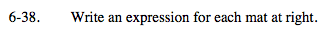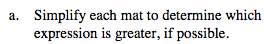Cancel out any zero pairs of tiles that you find.

How many of each tile is left from each side? Use this information to write an expression for each mat.

Mat A has the expression x + 3 since there is one x-tile and 3 unit tiles. Mat B has the expression 2x + 3. Now remove any matched sets from both sides.

We are left with:
Mat A equals 1
Mat B equals x
Since we don't know the value of x, we do not know which Mat is greater.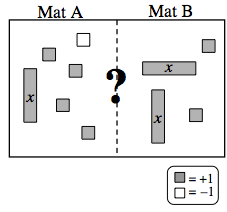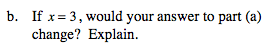Which Mat is greater when you substitute 3 for x?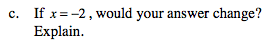Which Mat is greater when you substitute −2 for x?

Explore the problem with the eTool below.
Click the link at right for the full version of the eTool: MC2 6-38 HW eTool